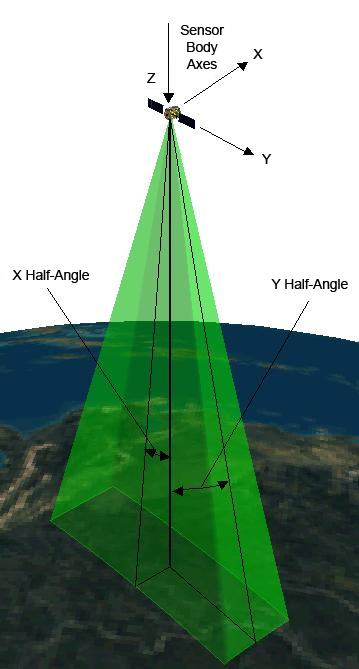agi.foundation.geometry.shapes
(agi.foundation.core-2023r1.jar)

## Class RectangularPyramid

• All Implemented Interfaces:
IServiceProvider

```public class RectangularPyramid
extends SensorFieldOfView```
A sensor volume with a rectangular cross section and a radial range limit. The half angles define the outer boundaries of the volume, and the radial range closes the volume, forming a sensor volume in the shape of a rectangular pyramid.

The vertex of the volume is located at the origin and the sensor principal direction is along the positive z-axis. Half angles measured from the principal direction and in the direction of the x-axis and y-axis define the extent of the rectangular cross section. The range limit measured radially from the origin closes the volume.• ### Constructor Summary

Constructors
Constructor and Description
`RectangularPyramid()`
Constructs a volume with a default definition, in which the `XHalfAngle` (`get` / `set`) and the `YHalfAngle` (`get` / `set`) are equal to `Constants.HalfPi` radians.
```RectangularPyramid(double xHalfAngle, double yHalfAngle)```
Constructs a volume of infinite radius with the provided parameters.
```RectangularPyramid(double xHalfAngle, double yHalfAngle, double radius)```
Constructs a volume of limited radius with the provided parameters.
• ### Method Summary

All Methods
Modifier and Type Method and Description
`boolean` `encloses(Cartesian point)`
Indicates if the provided point is inside the volume.
`EnclosureDescriptionCollection` `getEnclosureDescriptions()`
Gets the list of descriptions for the enclosure functions for this solid.
`boolean` `getIsConnected()`
Gets a value indicating whether the figure is connected.
`SensorProjection` ```getProjection(Ellipsoid ellipsoid, KinematicTransformation ellipsoidToSensorTransformation, SensorProjectionOptions projectionOptions)```
Gets the projection of the sensor onto the front surface of a specified ellipsoid and onto a plane at a specified distance in space.
`double` `getXHalfAngle()`
Gets the half angle measured from the principal direction and in the direction of the x-axis.
`double` `getYHalfAngle()`
Gets the half angle measured from the principal direction and in the direction of the y-axis.
`protected void` `onRadiusChanged()`
Called after the `Radius` (`get` / `set`) property changes.
`void` `setXHalfAngle(double value)`
Sets the half angle measured from the principal direction and in the direction of the x-axis.
`void` `setYHalfAngle(double value)`
Sets the half angle measured from the principal direction and in the direction of the y-axis.
• ### Methods inherited from class agi.foundation.geometry.shapes.SensorFieldOfView

`getProjection, getRadius, getService, setRadius`
• ### Methods inherited from class java.lang.Object

`clone, equals, finalize, getClass, hashCode, notify, notifyAll, toString, wait, wait, wait`
• ### Constructor Detail

• #### RectangularPyramid

```public RectangularPyramid(double xHalfAngle,
double yHalfAngle)```
Constructs a volume of infinite radius with the provided parameters.
Parameters:
`xHalfAngle` - The half angle measured from the principal direction and in the direction of the x-axis.
`yHalfAngle` - The half angle measured from the principal direction and in the direction of the y-axis.
• #### RectangularPyramid

```public RectangularPyramid(double xHalfAngle,
double yHalfAngle,
Constructs a volume of limited radius with the provided parameters.
Parameters:
`xHalfAngle` - The half angle measured from the principal direction and in the direction of the x-axis.
`yHalfAngle` - The half angle measured from the principal direction and in the direction of the y-axis.
`radius` - The radial limit of the volume. This is the maximum range at which the sensor can detect a target.
• ### Method Detail

• #### getIsConnected

`public boolean getIsConnected()`
Gets a value indicating whether the figure is connected.
Specified by:
`getIsConnected` in class `Figure`

`protected void onRadiusChanged()`
Called after the `Radius` (`get` / `set`) property changes.
Overrides:
`onRadiusChanged` in class `SensorFieldOfView`
• #### getXHalfAngle

`public final double getXHalfAngle()`
Gets the half angle measured from the principal direction and in the direction of the x-axis.
Throws:
`ArgumentOutOfRangeException` - Thrown when the half angle is less than or equal to zero or greater than `Constants.HalfPi` radians.
• #### setXHalfAngle

`public final void setXHalfAngle(double value)`
Sets the half angle measured from the principal direction and in the direction of the x-axis.
Throws:
`ArgumentOutOfRangeException` - Thrown when the half angle is less than or equal to zero or greater than `Constants.HalfPi` radians.
• #### getYHalfAngle

`public final double getYHalfAngle()`
Gets the half angle measured from the principal direction and in the direction of the y-axis.
Throws:
`ArgumentOutOfRangeException` - The half angle must be greater than zero and less than or equal to `Constants.HalfPi` radians.
• #### setYHalfAngle

`public final void setYHalfAngle(double value)`
Sets the half angle measured from the principal direction and in the direction of the y-axis.
Throws:
`ArgumentOutOfRangeException` - The half angle must be greater than zero and less than or equal to `Constants.HalfPi` radians.
• #### encloses

```public boolean encloses(@Nonnull
Cartesian point)```
Indicates if the provided point is inside the volume.

The `point` parameter must be expressed in the same coordinate frame that the volume is defined in.

Specified by:
`encloses` in class `Solid`
Parameters:
`point` - The point to evaluate.
Returns:
`true` if the point is inside the volume and `false` if the point lies on the boundary or outside of the volume.
• #### getEnclosureDescriptions

`public EnclosureDescriptionCollection getEnclosureDescriptions()`
Gets the list of descriptions for the enclosure functions for this solid.
Specified by:
`getEnclosureDescriptions` in class `Solid`
Returns:
The list of enclosure descriptions.
• #### getProjection

```public SensorProjection getProjection(Ellipsoid ellipsoid,
@Nonnull
KinematicTransformation ellipsoidToSensorTransformation,
SensorProjectionOptions projectionOptions)```
Gets the projection of the sensor onto the front surface of a specified ellipsoid and onto a plane at a specified distance in space.
Specified by:
`getProjection` in class `SensorFieldOfView`
Parameters:
`ellipsoid` - The ellipsoid on which the sensor is to be projected.
`ellipsoidToSensorTransformation` - The transformation from the reference frame of the ellipsoid to the reference frame of the sensor.
`projectionOptions` - The options controlling how the sensor is projected, or `null` to use defaults.
Returns:
The sensor projection.